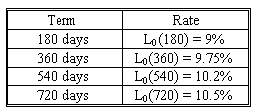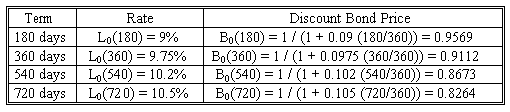### CFA Practice Question

There are 227 practice questions for this study session.

### CFA Practice Question

Quantum Electronics enters into a two-year \$20 million notional principal interest rate swap in which it promises to pay a fixed rate and receive payments at LIBOR. The payments are made every six months based on the assumption of 30 days per month and 360 days in a year. The term structure of LIBOR interest rates is given as follows:What should the fixed rate be?The annualized fixed rate would be R = (360/180) [(1 - 0.8264) / (0.9569 + 0.9112 + 0.8673 + 0.8264)] = 0.0975.

Thus, the rate would be 9.75%. The swap fixed payments would be \$20,000,000 x 0.0975 x 180/360 = \$975,000.